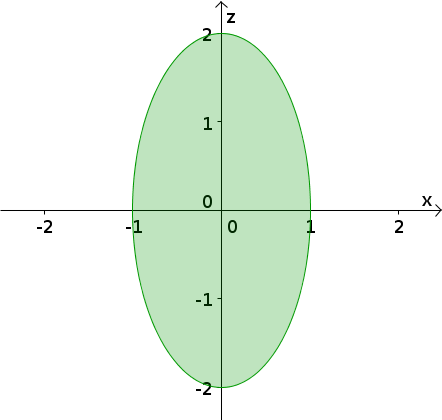# Math Insight

### Image: Triple integral ellipse shadowThe elliptical region $x^2+z^2/4 \le 1$ was computed as the shadow of the three-dimensional region bounded by $y=5-4 x^2-z^2$ and $y=x^2+z^2/4$ for the sun being at the positive $y$-axis.